Courses

# Signals And Systems & Microprocessors - MCQ Test - 2

## 20 Questions MCQ Test GATE ECE (Electronics) 2022 Mock Test Series | Signals And Systems & Microprocessors - MCQ Test - 2

Description
This mock test of Signals And Systems & Microprocessors - MCQ Test - 2 for Electronics and Communication Engineering (ECE) helps you for every Electronics and Communication Engineering (ECE) entrance exam. This contains 20 Multiple Choice Questions for Electronics and Communication Engineering (ECE) Signals And Systems & Microprocessors - MCQ Test - 2 (mcq) to study with solutions a complete question bank. The solved questions answers in this Signals And Systems & Microprocessors - MCQ Test - 2 quiz give you a good mix of easy questions and tough questions. Electronics and Communication Engineering (ECE) students definitely take this Signals And Systems & Microprocessors - MCQ Test - 2 exercise for a better result in the exam. You can find other Signals And Systems & Microprocessors - MCQ Test - 2 extra questions, long questions & short questions for Electronics and Communication Engineering (ECE) on EduRev as well by searching above.
*Answer can only contain numeric values
QUESTION: 1

### A sequence x(n) with the z-transform X(z) = z3 + 2z2 - 3z + z-1 - 4z-3 is applied as an input to a linear, time-invariant system with the impulse response h(n) = 28(n - 2) where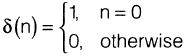The output at n = 2 is__________________________________

Solution:

The output y(n) can be drived as,
y(z) = I-1(z) X(z)

1.--1(z) = 2z-2

Y(z) = 2z-2 (z3 + 2z2 - 3z + z-1 - 4z-3) Y(z) 2(z + 2 - 3z-1 +

Taking inverse z-transform of Y(z) y(n) 2[6(n + 1) + 28(n) - 36(n - 1) +

(n - 3) -46(p - 511

At n = 2, y(2) = 0

QUESTION: 2

###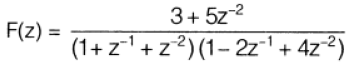then causal sequence f(n) is

Solution: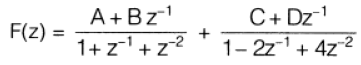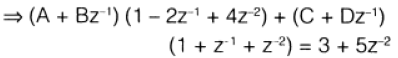On equating both sides we get. A 4- C = 3.

4A- 2B + C D = 5,

48 + D = 0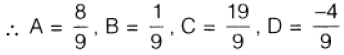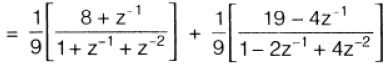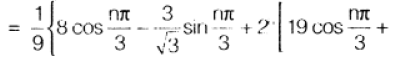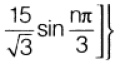QUESTION: 3

###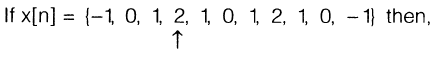Q. The value of X(eiπ) = ?

Solution:

Since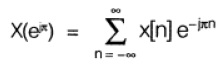∵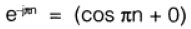= -1, when n = odd = +1, when rt = even

So,

X(e) = (2 +0+  2 + 0 +

[1 - 1 - 1 - 1 - 1 + 1]

4- 2 = 2

QUESTION: 4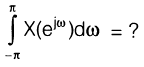Solution: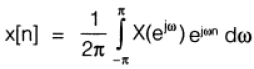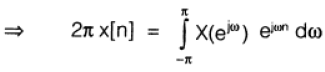at n 0, we have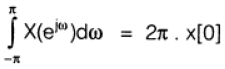= 2π X 2 = 4 π

QUESTION: 5

The Laplace transform of the waveform shown in the figure below is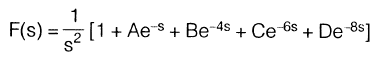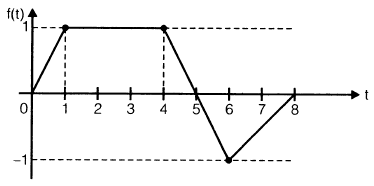Then what is the value of 'C'?

Solution:

From the waveform shown we have, f(t) t u(t) - (t - 1) u(t - 1) -

(t - 4) u(t - 4) + 1 .5(t - 6) u(t - 6)

- 1,5(t - 8) LAO - 8)

∴ F(s) = LT of f(t)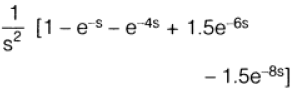Comparing this with the given Laplace transform and we get,

C = +1.5

QUESTION: 6

Fourier transform of the signal shown in figure below is,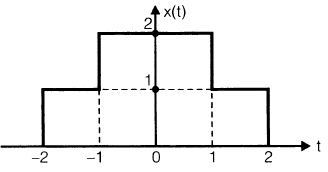Solution: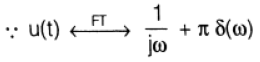Also, from the given plot we have

x(t) = u(t 2) + u(t + 1) - u(t - 1) - u(t - 2)

••• (I)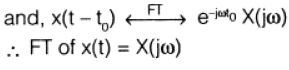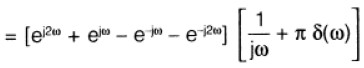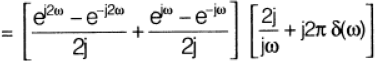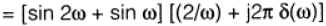QUESTION: 7

Answer Questions (22 and 23):

A DTS described by H(z) =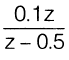when

excited by x[n] = cos(0.2 n + 10°) with discrete frequency Ω = 0.2.

Q. Find H(e) at Ω = 0.2 and is equals to

Solution:

For the given input x[n] cos 2n

response, y[n]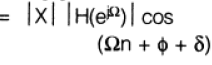Now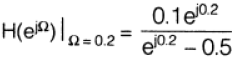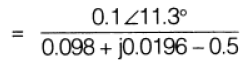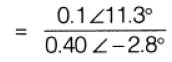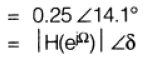QUESTION: 8

Answer Questions (22 and 23):

A DTS described by H(z) =when

excited by x[n] = cos(0.2 n + 10°) with discrete frequency Ω = 0.2.

Q. The steady state response y[n] is given by

Solution:

Now the steady state response

y[n] = 0.25 cos[0.2 n 10° + 14,1°) [n] = 0.25 cos[0.2 n +24.1°1

QUESTION: 9

Fourier analysis of waveform shown in figure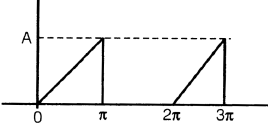Q. The waveform is

Solution:

wave form is neither odd nor even.

QUESTION: 10

Fourier analysis of waveform shown in figureQ.  In the waveform shown

1.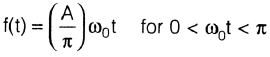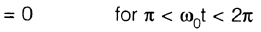2.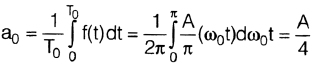3.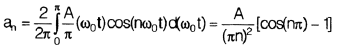4.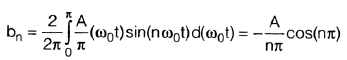5.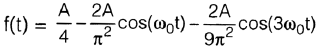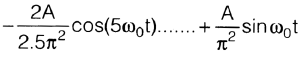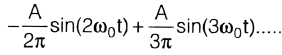Solution:
QUESTION: 11

Causal sequence f(n) if F(z) =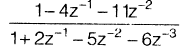, is given by

Solution: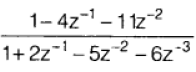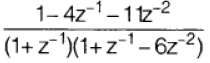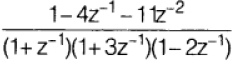........................(i)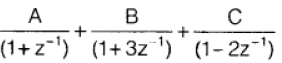........................(ii)

From 0) and 0) we get

A 1, B = 1, C = -1

Also we know, f(n) = Inverse z-transform of F(z)

f(n) = [(-1)n (-3)n - (2)n ] u (n)

QUESTION: 12

A signal x1(t) is having Fourier transform

Xi(co). Another signal x2(t) having Fourier transform X2(w) is related to Xi(co) by X2(w) = [1 +sg n(w)]Xi (co)

Value of x2(t) in terms of x1(t) will be

Solution: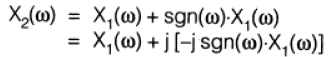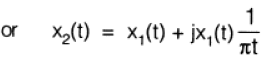QUESTION: 13

After some arithmetic operation flag register of 8085 microprocessor becomes BBH. The contents of the accumulator after the operation may be

Solution:

Flag registers in 808511P is as,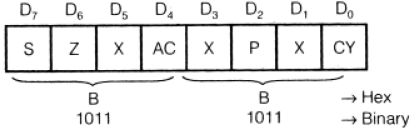Since, sign bit of 138.1-1. is 1 so, number is negative from the given choice only (c) or (d) gives the sign bit T.

Here, parity bit = 0 means total number of 1 's present is odd

So, only options (d) contains odd nu -r. her of 1's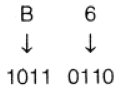Here five l's are present.

QUESTION: 14

The contents of register HL pair is 24FF H. Then the contents of register pair if, 1NR H and INR L operations are performed is

Solution:

∴  [H] <— 24
and [L] <— FF

INH H means increment the content of 'H' by 01.

∴          [H] <—  25

and       [L] <— FE

⇒ Content of H-L pair = 25FF H

L means increment the content of 'L'by 01.

∴    [H] <—  24

and    [L]   <— [FF] + 

i.e.  [L] <— 00

⇒ Content of IH-L pair = 2400 H

QUESTION: 15

If the HLT instruction of a 8085 microprocessor is executed then,

Solution:

HLT  →  is a 1-byte instruction.

→ processor stops executing and enters wait state

no register contents are affected.

the address bus and data bus are placed in high impedance state

QUESTION: 16

Line :                     MVI A,               B5H

MVI B,              OEH

XRI                   69H

ADD

ANI                    9BH

CPI                    9FH

STA                   3010 H

HLT

After execution of STA 3010H the status of Cy and Z flag will be

Solution:

Line    Cy        Z

1

2           0         0

3           0         0

4           0         0

0          0

6           1         0

7         1

QUESTION: 17

Three memory chips are of size 1 kB, 2 kB and 4 kB. Their address bus is 10 bits. The data bus sizes of chips are respectively

Solution:

∴ Memory size

= Add, bus size x Data bus size.

=(2)add bus line x.(Data bus size)

So, for 1 kB Chip.

Data bus size =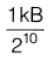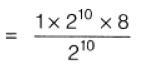For 2 kB chip,

Data bus size =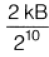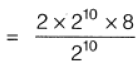16 bits

For 4 kB chip, Elata bus size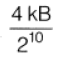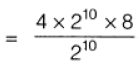= 32 bits

QUESTION: 18

An 8085 assembly language program is given below:

MV1C,           03H

LXI H,             2000 H

MOV             A, M

DCR

LOOP 1 : INX H

MOV B, M CMP B

JNC LOOP 2 MOV A, B

LOOP 2 : DCR C

JNZ LOOP 1 STA 2100 H HLT

Contents of memory location 2000: 18 H

2001 : 10H

2002 : 2BH

Q At the end of the program what will be the condition of carry and zero flags?

Solution:
QUESTION: 19

An 8085 assembly language program is given below:

MV1C,           03H

LXI H,             2000 H

MOV             A, M

DCR

LOOP 1 : INX H

MOV B, M CMP B

JNC LOOP 2 MOV A, B

LOOP 2 : DCR C

JNZ LOOP 1 STA 2100 H HLT

Contents of memory location 2000: 18 H

2001 : 10H

2002 : 2BH

What does the program do?

Solution:

Program is searching the value higher than the content of accumulator.

QUESTION: 20

Consider the given instructions of program for the delay register.

MVI           C, FFH

LOOP: DCR              C

JNZ           LOOP

Assume clock frequency of the system is 2 MHz.

Q.  The total T-states for this above programme is given by

Solution:

MVI C, FFH  →  7 T-states

LOOP: DCR C  → 5 T-states

JNZ LOOP → 10 T-states